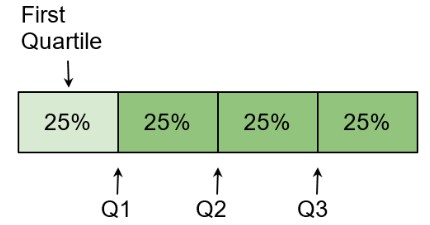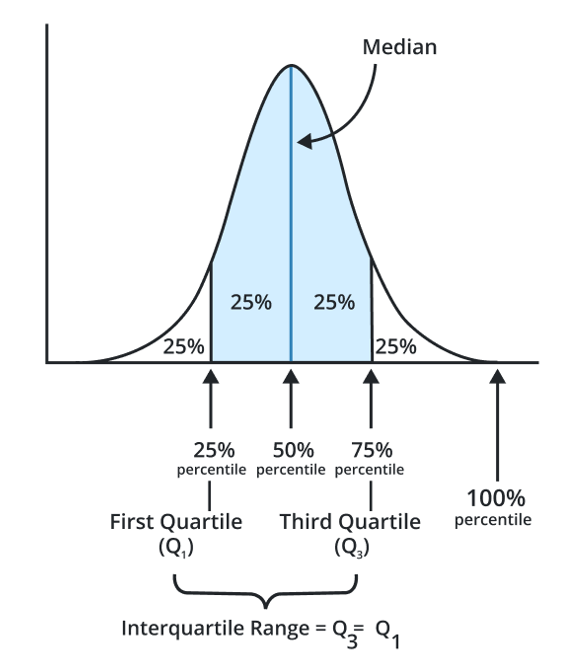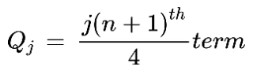# Quartile Calculator

Enter the numbers separated by commas in the quartile calculator and click calculate to find Q1, Q2, Q3, and IQR.

Give Us Feedback

## Quartile Calculator

Quartile calculator is an online tool that can find:

• First Quartile (Lower)
• Median (2nd Quartile)
• Third Quartile (Upper)
• Interquartile Range (IQR)

In this post, you will learn about quartiles and how to find them.

## Quartiles - Definition

Quartiles are three such points that divide a group into four equal parts. Each quartile accounts for a separate fourth part.### First Quartile (Q1)

It is the lower quartile which is the 25th percentile of the group. It is the point that divides the group in a ratio of 1:3.

### Second Quartile (Q2)

It is the central quartile which is the 50th percentile of the data. It is the point that divides the group in a ratio of 2:2. It is also called the median.

### Third Quartile (Q3)

It is the upper quartile which is the 75th percentile of the group. It is the point that divides the group in a ratio of 3:1.

Note: A group is divided into 100 percentiles.## Quartile Formulas

There is a general quartile formula that is adjusted according to the need for calculating a particular quartile. This formula is:Where ’J’ is the number of quartiles i.e. 1,2,3.

### Interquartile range formula

IQR = Q3 - Q1

Where Q3 and Q1 are the third and first quartiles respectively.

## How to find Quartiles?

Example

For the following set of data, find the 1st and 3rd quartiles. Also, find IQR.

3, 1, 6, 9, 12, 15, 18

Solution:

Step 1: Arrange the values in ascending order.

1, 3, 6, 9, 12, 15, 18

Step 2: Put the values in the quartile formula for the first quartile.

Q1 = 1(n+1)th/4 term

Q1 = 1(7+1)th/4 term

Q1 = (8)th/4 term

Q1 = 2nd term

2nd term is 3. So,

Q1 = 3

Step 3: Put the values in the quartile formula for the third quartile.

Q3 = 3(n+1)th/4 term

Q3 = 3(7+1)th/4 term

Q3 = 24th/4 term

Q3 = 6th term

6th term is 15. So,

Q3 = 15

Step 4: Put these values in the interquartile formula or use the IQR calculator above.

IQR = Q3 - Q1

IQR = 15 - 3

IQR = 12

### Math Tools